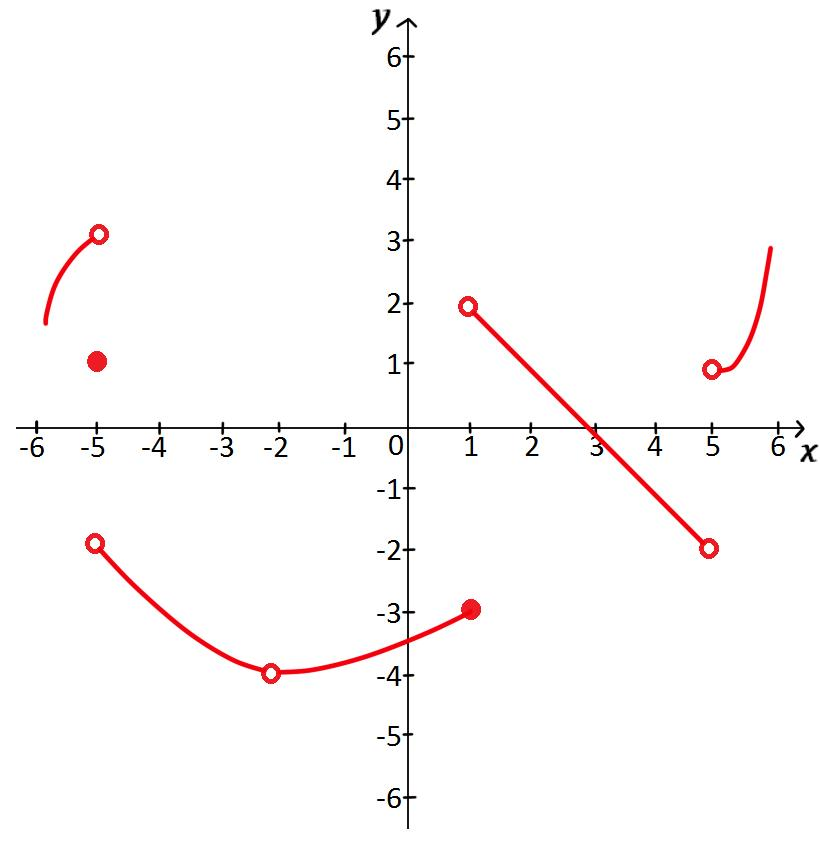# Finding limits from graphs

### Finding limits from graphs

Limit is an important instrument that helps us understand ideas in the realm of Calculus. In this section, we will learn how to find the limit of a function graphically using one-sided limits and two-sided limits.

#### Lessons

DEFINITION:
left-hand limit: $\lim_{x \to a^-} f(x) = L$
We say "the limit of f(x), as x approaches a from the negative direction, equals L".
It means that the value of f(x) becomes closer and closer to L as x approaches a from the left, but x is not equal to a.

DEFINITION:
right-hand limit: $\lim_{x \to a^+} f(x) = L$
We say "the limit of f(x), as x approaches a from the positive direction, equals L".
It means that the value of f(x) becomes closer and closer to L as x approaches a from the right, but x is not equal to a.

DEFINITION:
$\lim_{x \to a} f(x) = L$ if and only if $\lim_{x \to a^+} f(x) = L$ and $\lim_{x \to a^-} f(x) = L$
• 1.
For the function f whose graph is shown, state the following:a)
$\lim_{x \to -5^-} f(x)$
$\lim_{x \to -5^+} f(x)$
$\lim_{x \to -5} f(x)$
$f(-5)$

b)
$\lim_{x \to -2^-} f(x)$
$\lim_{x \to -2^+} f(x)$
$\lim_{x \to -2} f(x)$
$f(-2)$

c)
$\lim_{x \to 1^-} f(x)$
$\lim_{x \to 1^+} f(x)$
$\lim_{x \to 1} f(x)$
$f(1)$

d)
$\lim_{x \to 4^-} f(x)$
$\lim_{x \to 4^+} f(x)$
$\lim_{x \to 4} f(x)$
$f(4)$

e)
$\lim_{x \to 5^-} f(x)$
$\lim_{x \to 5^+} f(x)$
$\lim_{x \to 5} f(x)$
$f(5)$